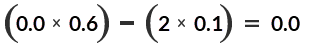An adaptive question is authored with multiple sections that are presented to a student one at a time to moderate the student's progression through a specific question.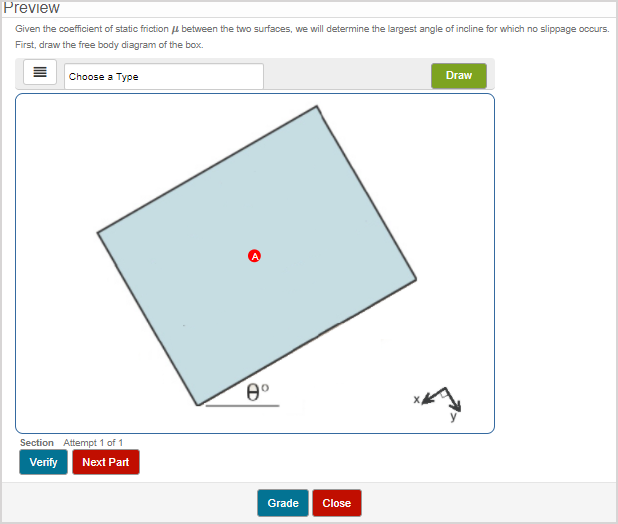TIP: You can also create adaptive assignments where a student's progression through a series of questions is moderated. Check out Create an adaptive assignment.

Each adaptive section within a question contains one or more response areas.

IMPORTANT: Adaptive sections are supported by any response area that's automatically graded by Möbius (Example — the true or false response area). Response areas that require manual grading (Exampleessay, document upload, and some Math App response areas) can't be used in adaptive questions.

The student's progression through each adaptive section of the question is determined by their performance on each previous section.

You can configure the adaptive section properties to moderate this progression (Example — the student could be allowed to work through a question step-by-step, or the student could be allowed another chance at the question with reduced credit if they initially submitted an incorrect response, or the student is permitted to attempt a simpler version of the question if they submit an incorrect before retrying the original question, etc.).

Adaptive questions are authored similarly to regular questions using the Question Editor, with the following additional features:

• Response areas are grouped into adaptive sections
• Properties are defined for each individual adaptive section
• There's a one-way workflow such that students can't return to previous sections within the adaptive question

The properties that you can define for each adaptive section are:

• The number of attempts the student is allowed at the section's response area(s)
• Whether the student can skip the section
• The passing score required for that section to be marked as correct
• Whether there's a penalty for using extra attempts
• The weighting of the section
• Whether the correct answer is displayed for the section after completion
• Whether the section remains visible to the student after completion
• If the question is considered complete once the section being attempted is complete

TIP: Check out step 5 in the To author an adaptive question section of this help topic for details on defining the adaptive section properties.

TIP: You can design different adaptive question layouts by customizing the properties of individual adaptive sections. Check out Configure different adaptive question layouts for ideas of how to apply these properties in different combinations for your adaptive question.

NOTE: Using an adaptive question in an activity with a time limit applied does permit the student to complete the entire adaptive question even after the time limit has expired. However, the student won't be able to navigate to another question once the time limit has expired. Check out Define assignment properties or Define lesson properties for more information on activity time limits.

## To author an adaptive question

1. Navigate to the Question Editor as if you were going to author a regular, non-adaptive question.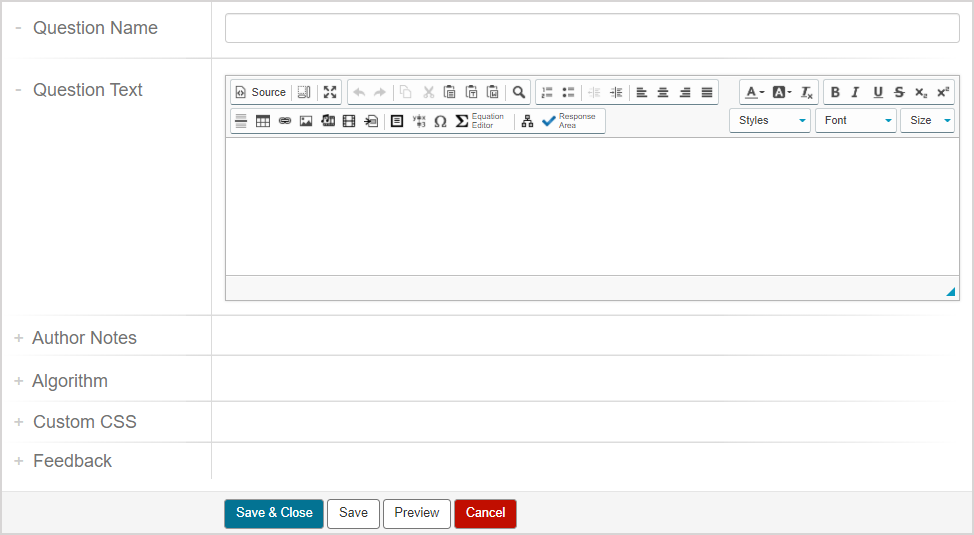TIP: Check out Author a question for reminders on how to access the Question Editor.

1. Author your question statement and all response areas for the question.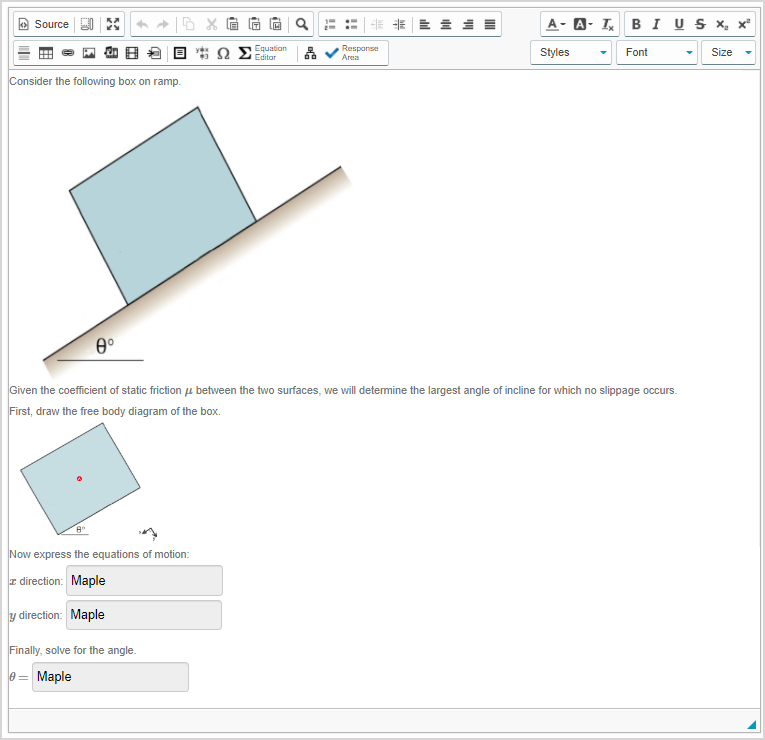TIP: An adaptive question can still have the following features, just like a non-adaptive question:NOTE: The adaptive section separator will be inserted as a gray divider to the right of your cursor and on the next row within your question. Each adaptive section is comprised of all of the preceding content from the adaptive section separator until the preceding separator (or beginning of the question if it's inserted as the first adaptive section separator).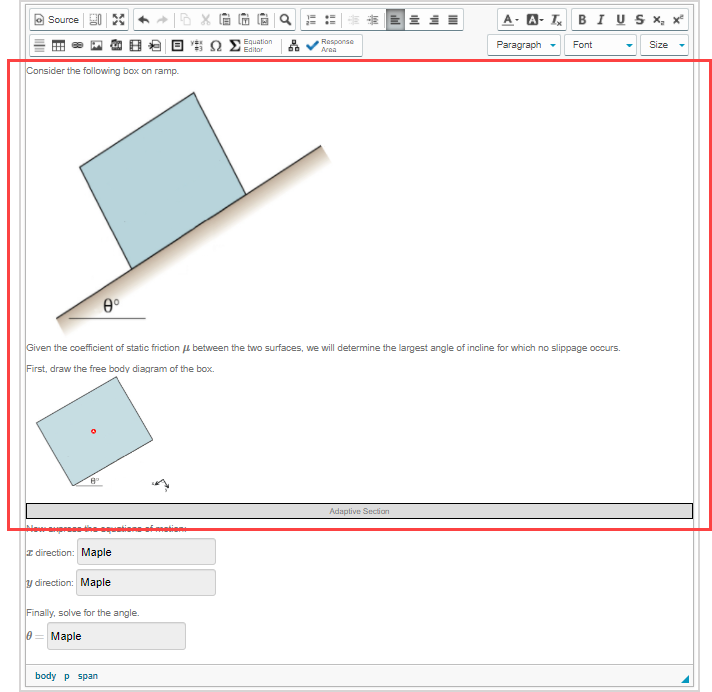1. Click Insert/Edit Adaptive Section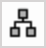from the Visual Editor toolbar to insert an adaptive section separator.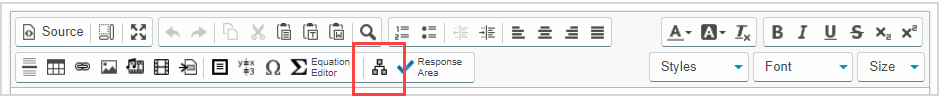1. Define the properties for the inserted adaptive section. There are three groups of properties to define for an adaptive section in the Edit Adaptive Section window: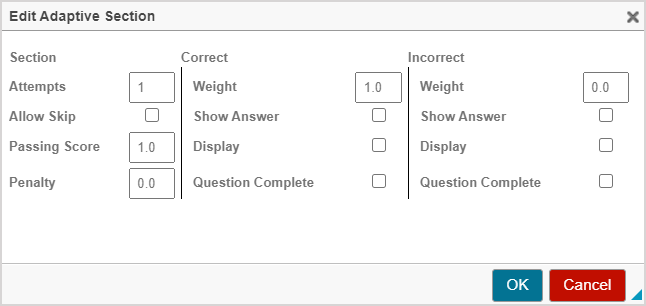• Section properties apply to the overall attempt at the adaptive section:
Section property Default setting Description
Attempts 1

Maximum number of allowed attempts at all response areas within the adaptive section.

Must be a positive integer.

NOTE: The number of allowed attempts applies to all response areas contained within the adaptive section. Example — Consider an adaptive section with three response areas. If a student enters a response into each response area and clicks Verify, that counts as one attempt. However, if a student only decides to enter a response to one of the three response areas and clicks Verify, that still counts as using one of their attempts.

Allow Skip Disabled

Enabled — The student can click the Next Part button to proceed to the next adaptive section (without entering a response or clicking Verify) and all remaining attempts at the skipped section are lost (their attempt is treated as if it's their last available attempt at the adaptive section).

NOTE: A student's entered response is still graded if Next Part is clicked instead of Verify. If no response is submitted and Next Part is clicked instead of Verify, it's treated as if the student submitted an incorrect response.

NOTE: If the student clicks Next Part and the question is considered complete if an incorrect response (or no response) is entered, the student won't be taken to the next section of the question and their attempt at the question is considered as complete.

Disabled — The Next Part button isn't visible and the student must complete all response areas in the section and click Verify to continue.

Passing Score 1.0

Section grade — A normalized value arrived at using the relative weightings of all response areas within an adaptive section.

NOTE: All response areas within an adaptive section are authored with a relative weight that's normalized to equal a value between 0.0 and 1.0. Example — An adaptive section with two response areas both with relative weights of 1 are normalized such that both response areas are worth 0.5 of the adaptive section's grade. If a student only gets one of the response areas correct, their section grade is 0.5.

The section grade is then compared to the Passing Score.

If the section grade is equal to or greater than the defined Passing Score, the response is considered correct and the grade awarded for the section is calculated using the Correct Response calculation.

If the section grade is less than the defined Passing Score, the response is considered incorrect and, if no available attempts remain (or all remaining attempts have been lost), the grade awarded is calculated using the Incorrect Response calculation.

TIP: Check out the Grading details for adaptive questions section of this help topic for more information on the Correct Response and Incorrect Response calculations.

Penalty 0.0

Determines the penalty to apply for each additional attempt at the section when more than one attempt is permitted for the section.

Must be an value between 0.0 and 1.0.

NOTE: When a penalty value is defined and the student can click Next Part, their grade for the section is calculated by multiplying one less than the total allowed attempts at the section by the penalty value. Check out the Grading details for adaptive questions section of this help topic for more information on how the penalty value affects the student's grade calculation.

• Correct properties apply to what happens when the student's section grade is considered correct based on the Passing Score:
Correct property Default setting Description
Weight 1.0

Relative weighting of the adaptive section in the overall question if the section is answered correctly.

Must be a value between 0.0 and 1.0.

IMPORTANT: Be sure that any combination of adaptive section grades achieved by your students upon question completion doesn't exceed 1.0.

Enabled — The correct answer is displayed when the section is completed and answered correctly.

Disabled — The correct answer isn't displayed when the section is completed and answered correctly.

Display Disabled

Enabled — The section remains visible to the student after it's completed and answered correctly.

Disabled — The section is no longer visible to the student after it's completed and answered correctly.

Question Complete Disabled

Enabled — The question is considered complete when this specific section is completed and answered correctly.

Disabled — The question isn't considered complete and the student is navigated to the next question section when this specific section is completed and answered correctly.

TIP: Be sure to select the Question Complete check box for the last adaptive section within your question so that the student is able to exit the question and continue to the next part of their activity.

• Incorrect properties apply to what happens when the student's section grade is considered incorrect based on the Passing Score:
Incorrect property Default setting Description
Weight 0.0

Relative weighting of the adaptive section in the overall question if the section is answered incorrectly.

Must be a value between 0.0 and 1.0.

IMPORTANT: Be sure that any combination of adaptive section grades achieved by your students upon question completion doesn't exceed 1.0.

Enabled — The correct answer is displayed when the section is completed and answered incorrectly.

Disabled — The correct answer isn't displayed when the section is completed and answered incorrectly.

Display Disabled

Enabled — The section remains visible to the student after it's completed and answered incorrectly.

Disabled — The section is no longer visible to the student after it's completed and answered incorrectly.

Question Complete Disabled

Enabled — The question is considered complete when this specific section is completed and answered incorrectly.

Disabled — The question isn't considered complete and the student is navigated to the next question section when this specific section is completed and answered incorrectly.

TIP: Be sure to select the Question Complete check box for the last adaptive section within your question so that the student is able to exit the question and continue to the next part of their activity.

TIP: Check out Configure different adaptive question layouts for ideas of how to apply these properties in different combinations for your adaptive question.

1. Click OK to insert the adaptive section separator.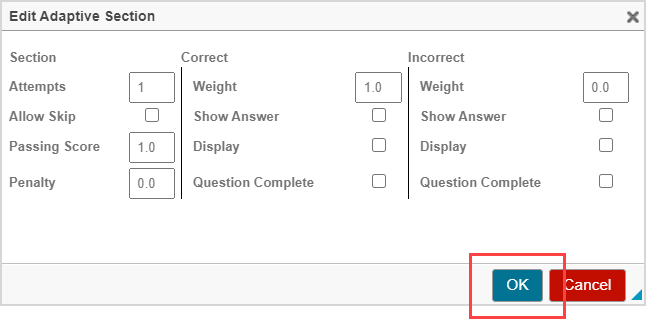1. Perform steps 3 to 6 as many times as needed for the number of adaptive sections you want to use in your question. Example — This question has three adaptive sections with a total of four response areas (a free body diagram response area and three Maple-graded response areas):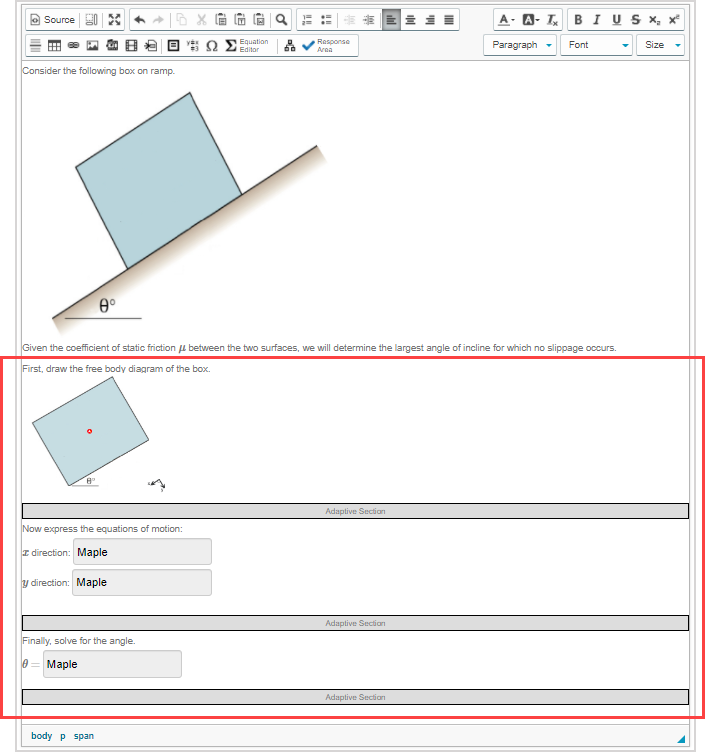TIP: Alternatively, you can add adaptive sections as you progress through authoring each response area.

1. Click Save to save your new question. Your new question is now stored in the Questions pane of the Content Repository.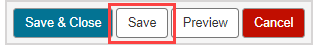TIP: Click the save iconif you're creating this question from within an Activity Editor. This saves your question to the activity and the Content Repository.

TIP: Be sure to preview your adaptive question to ensure that it behaves as expected.

• Each adaptive section having its own defined weighting based on correct or incorrect responses
• Applying grade penalties when more than one attempt is used at an adaptive section

The grade of an individual adaptive section is calculated using the following formulas:

• Correct Response calculation
• Incorrect Response calculation

NOTE: If the grade of the Correct Response or Incorrect Response calculation results in a negative value, the awarded grade defaults to 0.

The section grade is compared to the Passing Score to determine which calculation to use for the individual adaptive section.

NOTE: Section grade — A normalized value arrived at using the relative weightings of all response areas within an adaptive section.

### Correct Response calculation

A student's response is considered correct when the section grade is equal to or greater than the section's Passing Score.

The Correct Response calculation is then used to determine the grade for the individual adaptive section: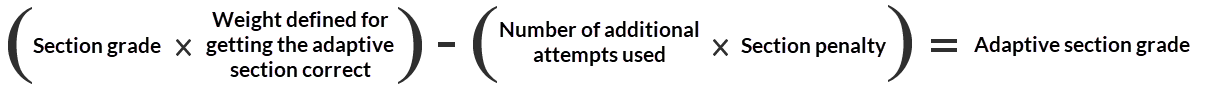NOTE: Here are some examples of how the Correct Response calculation is used:

Example — Consider the following scenario:

• An adaptive section has two response areas (both with a relative weight of 1), three allowed attempts, a passing score of 1.0, and a penalty of 0.1
• The adaptive section has the correct response weighted as 0.8
• The student:
• Achieved a section grade of 1.0 by answering both response areas correctly
• Used only one attempt
• Therefore receives an individual adaptive section grade of 0.8 based on the Correct Response calculation because the section grade was equal to the Passing Score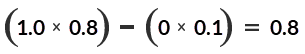Example — Consider the following scenario:

• An adaptive section has one response area, three allowed attempts, the student is permitted to skip the section using the Next Part button, a passing score of 1.0, and a penalty of 0.1
• The adaptive section has the correct response weighted as 0.8
• The student:
• Entered the correct response for the response area on their first attempt but clicked Next Part instead of Verify (the student's response that's entered into the Answer field is still graded without clicking Verify)
• Received the warning message that skipping the section will apply a penalty (if defined) and all remaining attempts become unavailable
• Therefore receives an individual adaptive section grade of 0.6 based on the Correct Response calculation because the section grade (even though Verify wasn't used) was equal to the Passing Score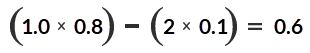### Incorrect Response calculation

A student's response is considered incorrect when the section grade is less than the section's Passing Score.

If no available attempts remain (or all remaining attempts have been lost), the Incorrect Response calculation is then used to determine the grade for the individual adaptive section: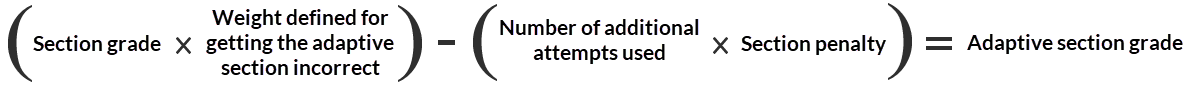NOTE: Here are some examples of how the Incorrect Response calculation is used:

Example — Consider the following scenario:

• An adaptive section has two response areas (both with a relative weight of 1), three allowed attempts, a passing score of 1.0, and a penalty of 0.1
• The adaptive section has the incorrect response weighted as 0.6
• The student:
• Used all three attempts
• Achieved a section grade of 0.5 on the final attempt by only answering one response area correctly
• Therefore receives an individual adaptive section grade of 0.1 based on the Incorrect Response calculation because section grade was less than the Passing Score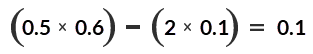Example — Consider the following scenario:

• An adaptive section has two response areas (both with a relative weight of 1), three allowed attempts, a passing score of 1.0, and a penalty of 0.1
• The adaptive section has the incorrect response weighted as 0.6
• The student:
• Used all three attempts
• Achieved a section grade of 0.0 on the final attempt by answering both response areas incorrectly
• Therefore receives an individual adaptive section grade of 0.0 based on the Incorrect Response calculation because section grade was less than the Passing Score